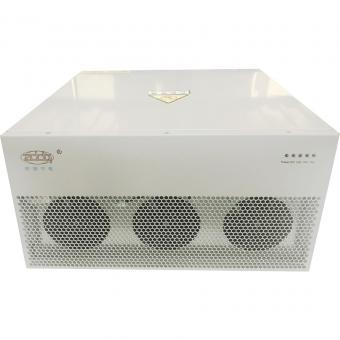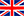English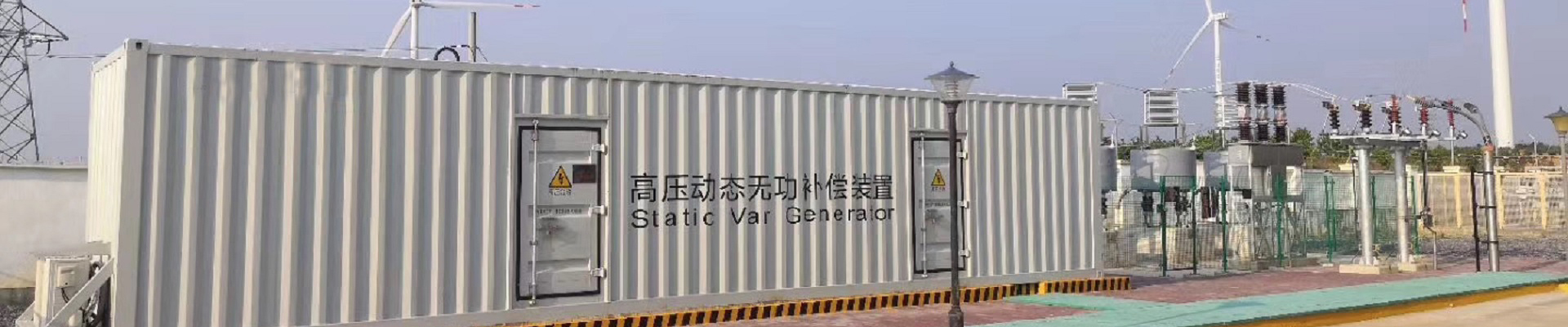ZDDQ Active Harmonic Filterpanel:200A,250A,300A,350A,400A,500A,600A.Standard or customerized cabinets.
•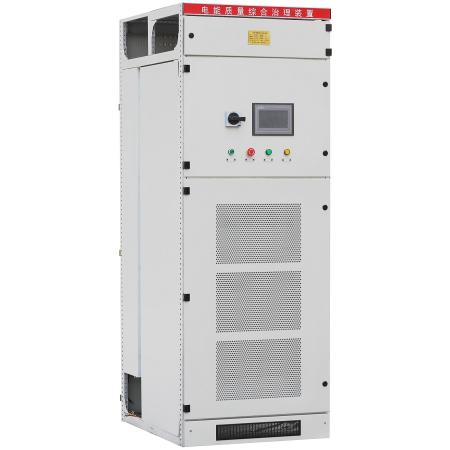#### Parallel 300A AHF active harmonic filter Panel

Active harmonic filter is connected in parallel with non-linear loads, and uses one set of CT to detect the load current. It calculates each order harmonic current by FFT algorithms in its DSP microchips, and then generates a compensating current with the same amplitude but opposite phase angles to the detected harmonic current, which cancels out the original load harmonics.Not only eliminates harmonic current from the load side, but it also mitigates harmonic voltage caused by harmonic currents. The AHF system can also improve power factor (PF) and correct load imbalances in the power system.
•#### 3P4L active power filter APF panels

Active Power filter is connected in parallel with non-linear loads, and uses one set of CT to detect the load current. It calculates each order harmonic current by FFT algorithms in its DSP microchips, and then generates a compensating current with the same amplitude but opposite phase angles to the detected harmonic current, which cancels out the original load harmonics.Not only eliminates harmonic current from the load side, but it also mitigates harmonic voltage caused by harmonic currents. The APF system can also improve power factor (PF) and correct load imbalances in the power system.
•#### Active harmonic filtering one-stop solution for power quality management harmonic filtering, reactive power compensation, load balancing

Active harmonic filter is connected in parallel with non-linear loads, and uses one set of CT to detect the load current. It calculates each order harmonic current by FFT algorithms in its DSP microchips, and then generates a compensating current with the same amplitude but opposite phase angles to the detected harmonic current, which cancels out the original load harmonics.Not only eliminates harmonic current from the load side, but it also mitigates harmonic voltage caused by harmonic currents. The AHF system can also improve power factor (PF) and correct load imbalances in the power system.
•#### 500A Rated compensation active harmonic filter cabinet

Active harmonic filter is connected in parallel with non-linear loads, and uses one set of CT to detect the load current. It calculates each order harmonic current by FFT algorithms in its DSP microchips, and then generates a compensating current with the same amplitude but opposite phase angles to the detected harmonic current, which cancels out the original load harmonics.Not only eliminates harmonic current from the load side, but it also mitigates harmonic voltage caused by harmonic currents. The AHF system can also improve power factor (PF) and correct load imbalances in the power system.
•#### Rated current compensation 200A AHF cabinets

Active harmonic filter is connected in parallel with non-linear loads, and uses one set of CT to detect the load current. It calculates each order harmonic current by FFT algorithms in its DSP microchips, and then generates a compensating current with the same amplitude but opposite phase angles to the detected harmonic current, which cancels out the original load harmonics.Not only eliminates harmonic current from the load side, but it also mitigates harmonic voltage caused by harmonic currents. The AHF system can also improve power factor (PF) and correct load imbalances in the power system.
•#### 400A Parallel APF active harmonic filter Panel supplies

Active harmonic filter is connected in parallel with non-linear loads, and uses one set of CT to detect the load current. It calculates each order harmonic current by FFT algorithms in its DSP microchips, and then generates a compensating current with the same amplitude but opposite phase angles to the detected harmonic current, which cancels out the original load harmonics.Not only eliminates harmonic current from the load side, but it also mitigates harmonic voltage caused by harmonic currents. The AHF system can also improve power factor (PF) and correct load imbalances in the power system.
1 2 3

a total of 3 pages

Hot Products
•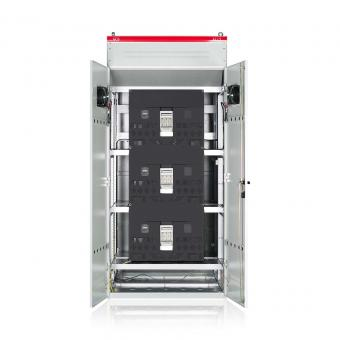#### Capacitor banks and harmonic filters. Low voltage

•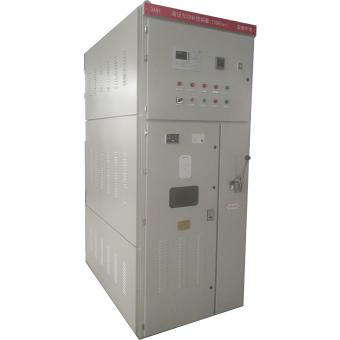#### 3.3KV Local reactive power compensation for motors,Arc Furnace

•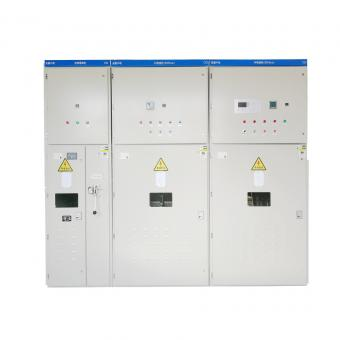#### Medium voltage Automatic Capacitor banks with harmonic filters

•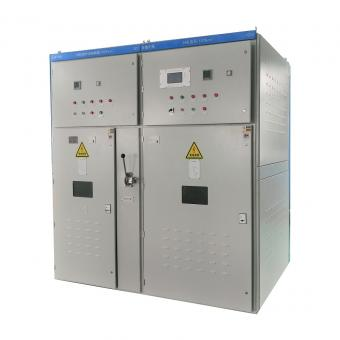#### High voltage Automatic Power Factor improvement plant for Motors VFDs

•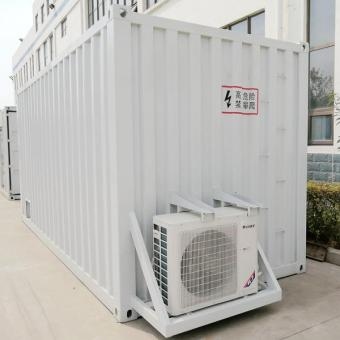#### High voltage static var generator HV SVG Statcom outdoor container

•#### Medium voltage outdoor Static Synchronous Compensator(STATCOM)

•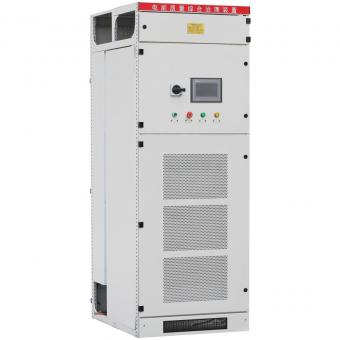•